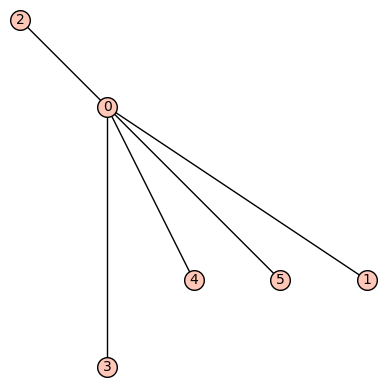# Does the set_embedding command have a bug?

We created a bipartite graph and called the set_embedding command by using a dictionary with vertices as keys and a list of neighboring vertices in clockwise order. The graph did not show the correct embedding. We expected the vertices to be in the correct clockwise order, but the order is not consistent. We also tried using the on_embedding command. Each time we run the code our vertices are randomly placed in different places.

EDIT:

#S0 and S1 are lists of lists

def Graf(S0, S1):
D={} #empty graph
G={} #edited graph that we add to
verts={}
#opening an empty graph
graph=Graph(D, multiedges=True)

#adds edges and vertices to the graph
#S0 and S1 are lists of lists
def Dessin(S0, S1):
numVertices = len(S0)+len(S1)

#creating dictionary G of vertices with neighboring edges in counterclockwise order
for i in range(numVertices):
G[i]=(S0+S1)[i]

verts[i]=[]
#iterate over black vertices
for i in range(len(S0)):
#iterate over edges connected to black vertices
for j in range(len(S0[i])):
#iterate over white vertices
for k in range(len(S1)):
#check if black and white vertices have an edge in common
if S0[i][j] in S1[k]:
#if they have an edge in common connect the vertices with the correct edge labeling

print('dictionary of neighboring edges:')
print(G)

#creating dictionary with vertices as keys and neighboring vertices in counterclockwise order
for i in range(numVertices):
for j in range(len(G[i])):
for k in range(numVertices):
if i!=k:
for l in range(len(G[k])):
if G[i][j] in G[k]:
if k not in verts[i]:
verts[i].append(k)
print("verts before flipped order:")
print(verts)

#flip order of vertices so that we're counterclockwise.
for i in range(len(verts)):
L=[]
for j in range(len(verts[i])):
L.append(verts[i][(len(verts[i]))-j-1])
verts[i]=L

#all the vertices in S0 are black, all the vertices in S1 are white
def color(S0, S1):
grey=[]
white=[]
for i in range(len(S0)):
grey.append(i)
for i in range(len(S1)):
white.append(i+len(S0))
return [grey,white]

#call and color the graph
Dessin(S0, S1)
color(S0, S1)
print("verts after flipped order:")
print(verts)

#keep a certain ordering
graph.set_embedding(verts)

edit retag close merge delete

Please edit your question: paste the relevant code, select it and press the 101010 button to indent it by four spaces (to get the proper formatting).

I am not sure whether our planar plot function respects the embedding or not :

sage: G = graphs.RandomTriangulation(10)
sage: G.plot(layout='planar')


But at least it displays a planar graph.

Please include a full code sample, since G, verts and graphare currently not defined. Also include a full code sample of how you want to call this function, what you expect as output, and what you get instead. (It should be possible to run it e.g. on https://sagecell.sagemath.org/) It is very difficult to help you otherwise.

Sort by » oldest newest most votedSetting the combinatorial embedding does not immediately affect how the graph is plotted. However, you can call one of the layout algorithms that use this combinatorial embedding, such as planar, with save_pos=True.

G = Graph([[0,1,2,3,5,4],[[0,1],[0,2],[0,3],[0,4],[0,5]]])
G.set_embedding({0: [1,2,3,4,5], 1 : , 2 : , 3:, 4:, 5:})
G.layout('planar', save_pos=True)
G.show()Indeed we get clockwise 1, 2, 3, 4, 5.

Edit: Obviously not clockwise but counterclockwise. I opened trac ticket #28152 for this issue.

more